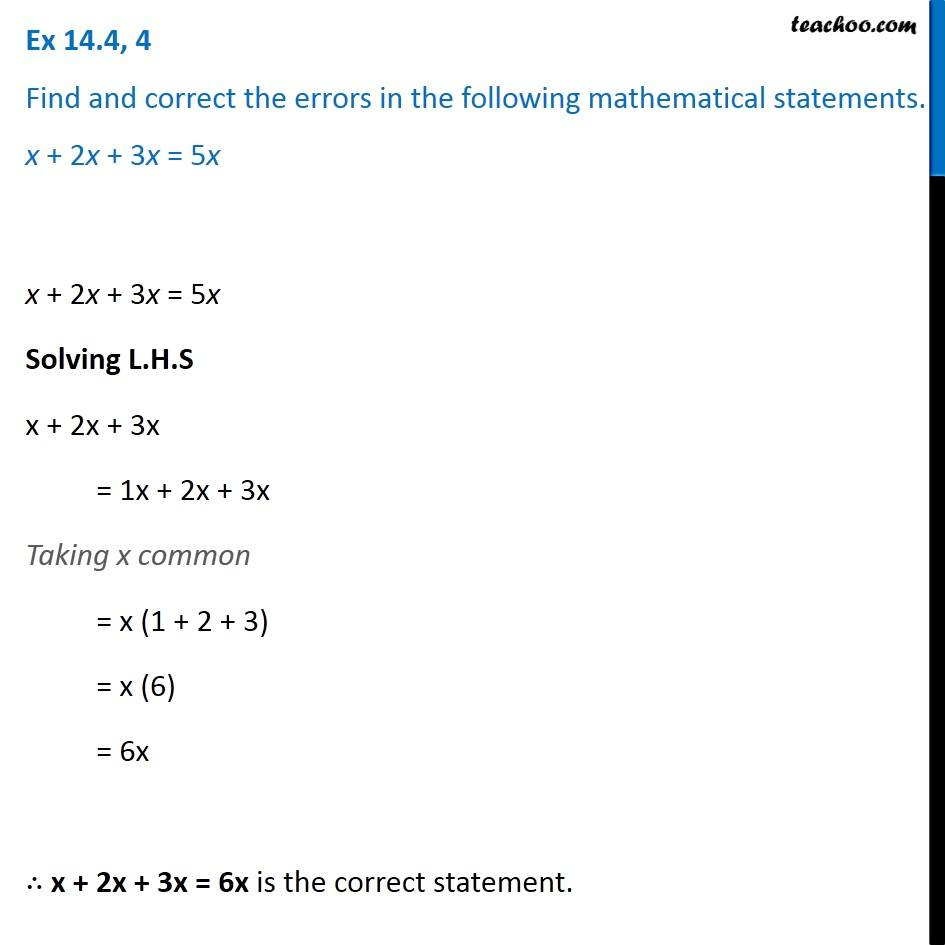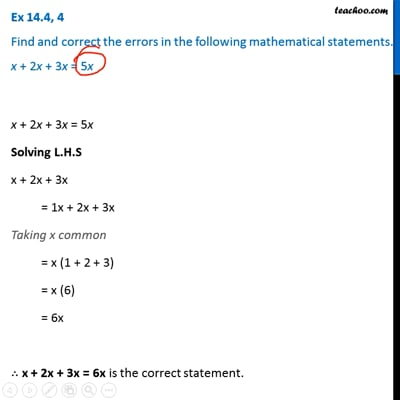Ex 14.4

Chapter 14 Class 8 Factorisation
Serial order wiseThis video is only available for Teachoo black users

Get live Maths 1-on-1 Classs - Class 6 to 12

### Transcript

Ex 14.4, 4 Find and correct the errors in the following mathematical statements. x + 2x + 3x = 5x x + 2x + 3x = 5x Solving L.H.S x + 2x + 3x = 1x + 2x + 3x Taking x common = x (1 + 2 + 3) = x (6) = 6x ∴ x + 2x + 3x = 6x is the correct statement.